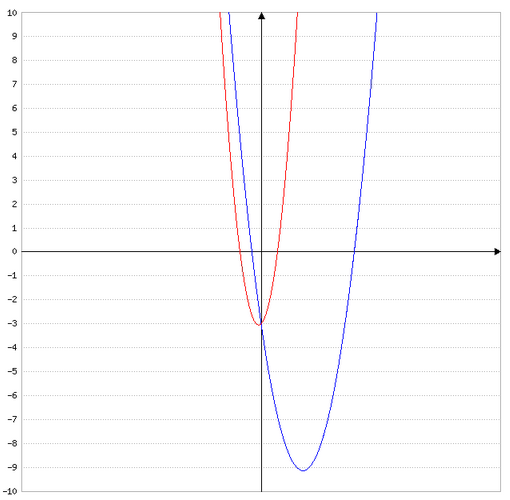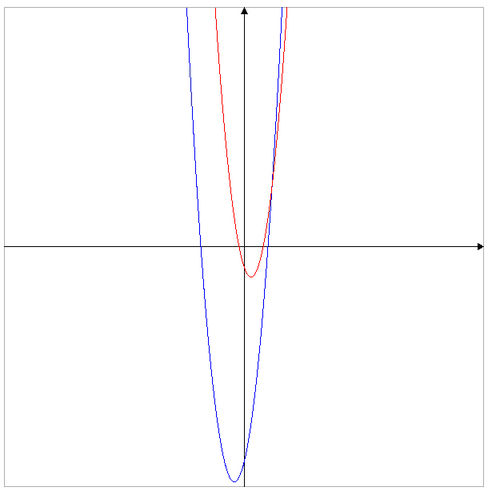# GRE Quantitative Comparison Tip #1 – Dealing with Variables

Here's the whole series of QC tips:

Tip #1: Dealing with Variables

Tip #2: Striving for Equality

Tip #3: Logic over Algebra

Tip #4: Comparing in Parts

Tip #5: Estimation with a Twist

We'll examine a useful strategy that can increase the effectiveness of the plug-in-numbers approach.

To set up today's strategy, please consider the following QC question:

 Column A Column B 2x^2 - 7x -3 5x^2 + x -3

A. The quantity in Column A is greater

B. The quantity in Column B is greater

C. The two quantities are equal

D. The relationship cannot be determined from the information given\blue{f_1(x) = 2x^2 - 7x -3}
\red{f_2(x) = 5x^2 + x -3}

Let's see: f_1(x) - f_2(x)

= (2x^2 - 7x -3) - (5x^2 + x -3)

= 3x^2 + 8x

= x(3x+8)

When x=0 \space or \space x=-\dfrac{3}{8}f_1(x) = f_2(x) = 0

It is obvious that if x \gt 0 then x(3x+8) \gt 0

It is obvious that if x = -1 then x(3x+8) \lt 0

You can approach the question algebraically, or by plugging in numbers.

Now, as I mentioned in the last post, the algebraic approach provides the most conclusive results. However, before I begin applying algebraic techniques, I should determine whether it may be faster to use the plug-in-numbers approach. I do this by asking the following question:

“Is there a nice value for the variable that makes the two columns equal?”

Notice that I said “nice.” Obviously, we don't want to spend 2 minutes looking for values of x that make the two columns equal. So, I'm going to consider the usual suspects: 1, 0 and -1, and perhaps some other numbers if they look obvious.

Now, when we examine the question above, we see that both expressions have a constant term (the term without any variables) of 3.

Since all of the other terms have x in them, we can make those other terms equal zero if we let x = 0. So, when x = 0, we get:

 Column A Column B 2(0)^2 - 7*(0) - 3 5(0)^2 + (0) - 3

When we evaluate this, we get:

 Column A Column B -3 -3

At this point—within a matter of seconds, and without any significant calculations—we already know that our answer must be either C (the two quantities are always equal), or D (the relationship cannot be determined from the information given).

All we need to do now is try another number. When we plug that new number, we know that if the two quantities are not equal, then the answer must be D. If the two quantities are still equal, then the answer is leaning towards C.

Now, which number should we try? Well, we should choose one that makes it easy to evaluate both sides.

How about 1? When x = 1, we get:

 Column A Column B 2(1)^2 - 7*(1) - 3 5(1)^2 + (1) - 3

When we evaluate this, we get:

 Column A Column B -8 3

Since the two columns are not equal when x=1, the correct answer is D.

There's a big advantage in looking for ways to make the two columns equal, rather than just plugging in numbers. Let's say you plug in a number, and it turns out that Column A is greater than Column B. At this point, we want to find a value for the given variable, such that Column B is greater than Column A. This will allow us to be certain that the answer is D.

Alternatively, if we find a value for the given variable, such that the two columns are equal, we now need only find a value for the given variable such that the two columns are not equal. This is a much easier task than looking for values that make one column greater than the other.

Okay, let's try another question:

 Column A Column B (x-2)(x-5)(x+9) (x+1)(x-4)(x-2)

A. The quantity in Column A is greater

B. The quantity in Column B is greater

C. The two quantities are equal

D. The relationship cannot be determined from the information given

f_1(x)=(x-2)(x-5)(x+9)

f_1(x)=(x+1)(x-4)(x-2)

When x=2, f_1(x)=f_2(x)=0

When x \not{=} 2, we can divide both columns by x-2 to get:

 Column A Column B (x-5)(x+9) (x+1)(x-4)

When we expand the function, we get:

 Column A Column B x^2 + 4x - 45 x^2 -3x - 4\blue{f_1(x) = x^2 + 4x - 45}
\red{f_2(x) = x^2 -3x - 4}

Let's see: f_1(x) - \gt f_2(x)

= (x^2 + 4x - 45) - (x^2 -3x - 4)

= 7x - 41

= 7(x - \dfrac{41}{7})

If x \not{=} 2 and x \gt \dfrac{41}{7}, then f_1(x) - \gt f_2(x).

If x \not{=} 2 and x \lt \dfrac{41}{7}, then f_1(x) - \lt f_2(x).

If we wanted to use the algebraic approach here, we'd have to expand each side and do a lot of work to solve the question.

Alternatively, if we ask the question, “Is there a nice value for the variable that makes the two columns equal?”, we can see that, when x = 2, both columns evaluate to be zero:

 Column A Column B (2-2)(2-5)(2+9) (2+1)(2-4)(2-2)

Since 2 - 2 = 0, both products will evaluate to be zero.

At this point, we need only find a value for x such that the two columns are not equal.

Well, a nice number to plug in for x is 5. When x=5, we get:

 Column A Column B (5-2)(5-5)(5+9) (5+1)(5-4)(5-2)

At this point, we can see that the Column A will evaluate to be zero, and Column B will not evaluate to be zero. We don't really care about which column is greater. All we care about is that the two columns are not equal, which means the answer is D.

So, when tackling QC questions involving variables, be sure to ask, “Is there a nice value for the variable that makes the two columns equal?”